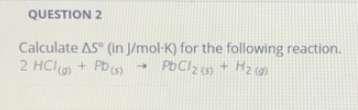# Problem: Calculate ΔS° compositefunction (in J/mol • K) for the following reaction. 2HCl (g) + Pb (s) → PbCl2 (s) + H2 (g)

###### FREE Expert Solution
98% (404 ratings)###### Problem Details

Calculate ΔS° compositefunction (in J/mol • K) for the following reaction.

2HCl (g) + Pb (s) → PbCl(s) + H(g)